﻿ 2016年门源<i>M</i><sub>S</sub> 6.4地震前后视应力变化文章快速检索
 地震地磁观测与研究2018, Vol. 39Issue (1): 53-59  DOI: 10.3969/j.issn.1003-3246.2018.01.0090

### 引用本文Liu Wenbang, Li Qilei. The variations of apparent stress before and after Menyuan MS 6.4 earthquake in 2016[J]. Seismological and Geomagnetic Observation and Research, 2018, 39(1): 53-59. DOI: 10.3969/j.issn.1003-3246.2018.01.009.

### 文章历史

2016年门源MS 6.4地震前后视应力变化

The variations of apparent stress before and after Menyuan MS 6.4 earthquake in 2016
Liu Wenbang, Li Qilei
Qinghai Earthquake Agency, Xining 810001, China
Abstract: Based on waveform date observed by the regional seismic netwok of Qinghai Province, we calculated source parameters of 184 ML ≥ 2.5 earthquakes occurred in research area from 2010 to 2016 and 150 ML ≥ 2.0 Menyuan aftershocks in 2016, analyzed temporal-spatial variations of apparent stress. The results show there is a positive correlation between apparent stress and magnitude. which increases with the increase of magnitude; The apparent stress of medium-small earthquakes before Menyuan M 6.4 earthquake change obviously. It may reflect the enhancement of regional stress field. The apparent stress showed significant increase before Menyuan ML5.0 earthquakes.
Key Words: Menyuan M 6.4 earthquake    source parameters    apparent stress
0 引言

1 震源动力学参数计算方法

 ${\sigma _{{\rm{app}}}} = \mu \frac{{{E_{\rm{S}}}}}{{{M_0}}}$ (1)

 $\mathit{\Omega }\left(f \right) = \frac{\mathit{\Omega }}{{1 + \frac{f}{{{f_{\rm{c}}}}}}}$ (2)

 ${M_0} = \frac{{4\pi {v^3}\mathit{\Omega }d}}{R}$ (3)

 $v\left(f \right) = \sqrt {v\left(f \right)_{\rm{Z}}^2 + v\left(f \right)_{{\rm{NS}}}^2 + v\left(f \right)_{{\rm{EW}}}^2}$ (4)

 ${E_{\rm{S}}} = 8\pi \rho \beta \int_0^\infty {v{{\left(f \right)}^2}{\rm{d}}f}$ (5)

 ${E_{\rm{S}}} = 8\pi \rho \beta \left\{ {\frac{1}{3}{{\left[ {2\pi {f_1}{\mathit{\Omega }_0}} \right]}^2}{f_1} + \int_{{f_1}}^{{f_2}} {v{{\left(f \right)}^2} + {{\left[ {2\pi {f_3}\mathit{\Omega }\left({{f_3}} \right)} \right]}^2}{f_3}} } \right\}$ (6)

 $\bar x{\rm{ = exp}}\left({\frac{1}{N}\sum\limits_{i = 1}^N {\ln \;{x_i}} } \right)$ (7)
 $\Delta x = \exp \left({\sqrt {\frac{1}{{N - 1}}{{\sum\limits_{i = 1}^N {\left[ {\ln \;{x_i} - \ln \;\bar x} \right]}^2 }}} } \right)$ (8)

2 数据资料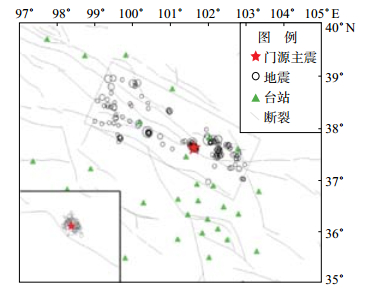图 1 研究区域2010—2016年地震及台站分布 Fig.1 Distribution of earthquakes and stations in the research area from 2010 to 2016
3 计算结果 3.1 震源动力学参数定标关系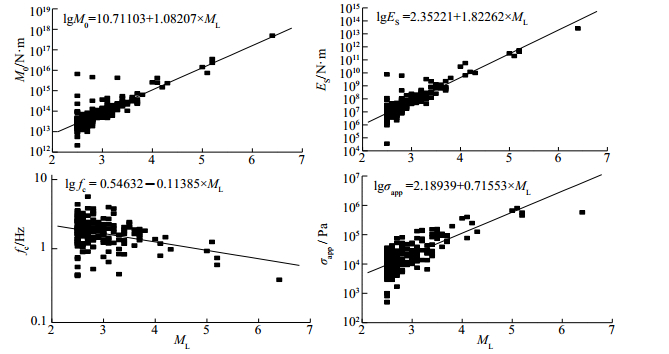图 2 研究区震源动力学参数与震级的定标关系 Fig.2 The scaling relationship between the seismic source dynamics parameter and the magnitude in the study area

 $\left(1 \right){\rm{地震矩与震级}}\;\;\;\lg {M_0} = 10.71103 + 1.08207{M_{\rm{L}}}$ (9)
 $\left(2 \right){\rm{辐射能量与震级}}\;\;\;\lg {E_{\rm{S}}} = 2.35221 + \begin{array}{*{20}{l}} {1.82262} \end{array}{M_{\rm{L}}}$ (10)
 $\left(3 \right){\rm{拐角频率与震级}}\;\;\;\lg {f_{\rm{c}}} = \begin{array}{*{20}{l}} {0.54632} \end{array} - \begin{array}{*{20}{l}} {0.11385} \end{array}{M_{\rm{L}}}$ (11)
 $\left(4 \right){\rm{视应力与震级}}\;\;\;\lg {\sigma _{{\rm{app}}}} = \begin{array}{*{20}{l}} {2.18939} \end{array}{\rm{ + }}\begin{array}{*{20}{l}} {0.71553} \end{array}{M_{\rm{L}}}$ (12)

3.2 研究区视应力变化特征

2016年门源6.4级地震发生在甘青交界的青海境内（图 1），据发震断层走向和地震台站分布，选取震中附近的矩形区域为研究区。青海区域地震台网对该区ML≥3.0地震有较完整记录。图 3给出研究区2010-2016年ML≥3.0地震的M-T关系、视应力及拐角频率随时间变化关系曲线。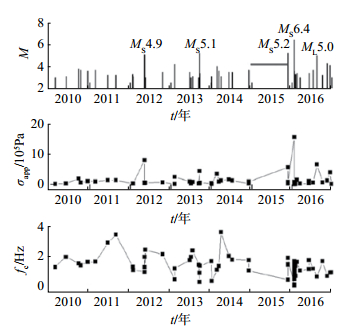图 3 研究区ML ≥ 3地震M—T、视应力及拐角频率随时间的变化关系 Fig.3 The change with time of M—T, apparent stress and corner frequency of earthquakes in the research area

M-T图可以发现，该时段内依次发生2012年5月11日甘肃肃南4.9级地震、2013年9月20日青海门源5.1级、2015年11月23日祁连5.2级及2016年1月21日门源6.4级等显著地震事件。在2015年11月23日祁连地震发生前，研究区域存在约1年的ML 3地震平静期，祁连5.2级地震将平静打破后2个月发生门源6.4级地震。对视应力、拐角频率进行对比分析，由视应力随时间的变化曲线发现，几次较大地震对应的视应力明显偏高，视应力存在起伏变化，但异常不明显。由拐角频率随时间变化的曲线发现，2011-2015年存在数次高频地震事件，ML 3地震平静后发生的地震，拐角频率较之前起伏较小，在一定程度上反映了区域应力存在积累-地震平静-发震的过程。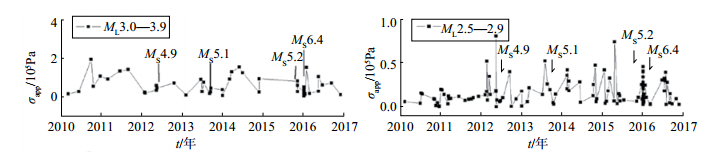图 4 研究区域ML 3.0—3.9、ML 2.5—2.9地震视应力随时间的变化 Fig.4 The change with time of apparent stress of ML 3.0—3.9 and ML 2.5—2.9 earthquakes in the research area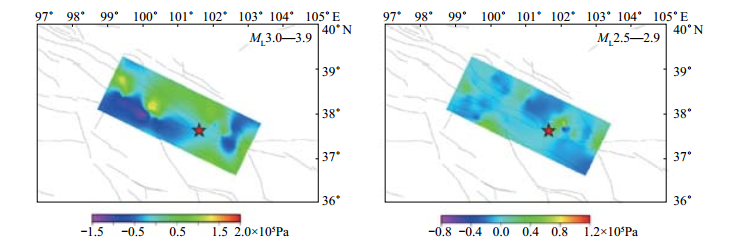图 5 门源6.4级地震前ML 3.0—3.9和ML 2.5—2.9震级段地震视应力空间分布 Fig.5 Spatial distribution of apparent stress of ML 3.0—3.9 and ML 2.5—2.9 earthquakes before Menyuan MS 6.4 in the research area
3.3 门源地震序列视应力变化特征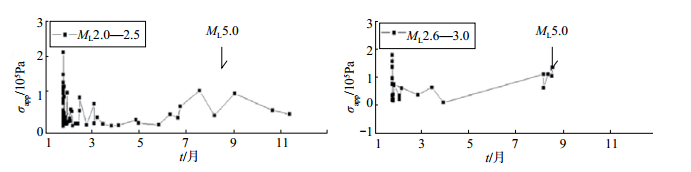图 6 2016年门源地震序列ML 2.0—2.5和ML 2.6—3.0震级段视应力随时间变化 Fig.6 The change with time of apparent stress of ML 2.0—2.5 and ML 2.6—3.0 Menyuan earthquakes in 2016
4 结论与讨论

（1）通过对地震序列震源参数与震级的定标关系进行分析，发现地震矩、地震辐射能量与震级存在较好的正相关性；拐角频率、视应力与震级存在较明显的相关性，拐角频率随震级增大而减小，视应力随震级增大而增大。

（2）研究区域在门源地震前存在约1年的ML 3地震平静，ML≥3.0地震拐角频率在地震平静之前起伏变化明显，门源地震后变化减小。研究区域ML 3.0-3.9和ML 2.5-2.9震级段地震视应力在门源地震前存在不同程度的起伏变化，反映了门源地震前区域应力场的增强。

（3）门源6.4级地震序列ML 2.0-2.5和ML 2.6-3.0震级段视应力表现出主震后短时间内发生的余震视应力较高、随时间逐渐降低的特点，但发生ML 5.0强余震前，小震视应力呈现了升高的变化过程，可能反映了震源区应力的增强。

 陈学忠, 李艳娥. 2006年7月4日河北文安MS 5.1地震前震中周围地区小震视应力随时间的变化[J]. 中国地震, 2007, 23(4): 327-336. 陈学忠, 王小平, 王林瑛, 等. 地震视应力用于震后趋势快速判定的可能性[J]. 国际地震动态, 2003(7): 1-4. 华卫, 陈章立, 郑斯华. 2008年汶川8.0级地震序列震源参数分段特征的研究[J]. 地球物理学报, 2009, 52(2): 365-371. 李国佑, 姚家骏. 2008年、2009年大柴旦两次6级地震序列地震视应力特征[J]. 地震地磁观测与研究, 2015, 36(3): 53-57. 李艳娥, 陈学忠. 1999年11月29日岫岩5.4级地震序列震源参数测定及标度关系分析[J]. 地震, 2007, 27(4): 59-67. 钱晓东, 李琼, 秦嘉政. 2007年宁洱6.4级地震序列视应力研究[J]. 地震研究, 2007, 30(4): 311-317. 吴忠良. 由宽频带辐射能量目录和地震矩目录给出的视应力及其地震学意义[J]. 中国地震, 2001, 17(1): 8-15. 吴忠良, 黄静, 林碧苍. 中国西部地震视应力的空间分布[J]. 地震学报, 2002, 24(3): 293-301. 杨志高, 张晓东. 首都圈地区地震视应力的计算及空间分布[J]. 地震, 2009, 29(4): 32-43. Archuleta R J, Cranswick E, Mueller C, et al. Source parameters of the 1980 Mammoth Lakes, California, earthquake sequence[J]. J Geophys Res, 1982, 87(B6): 4595-4607. DOI:10.1029/JB087iB06p04595 Wyss M, Brune J N. Seismic moment, stress, and source dimensions for earthquakes in the California Nevada Region[J]. J Geophys Res, 1968, 73(14): 4681-4694. DOI:10.1029/JB073i014p04681